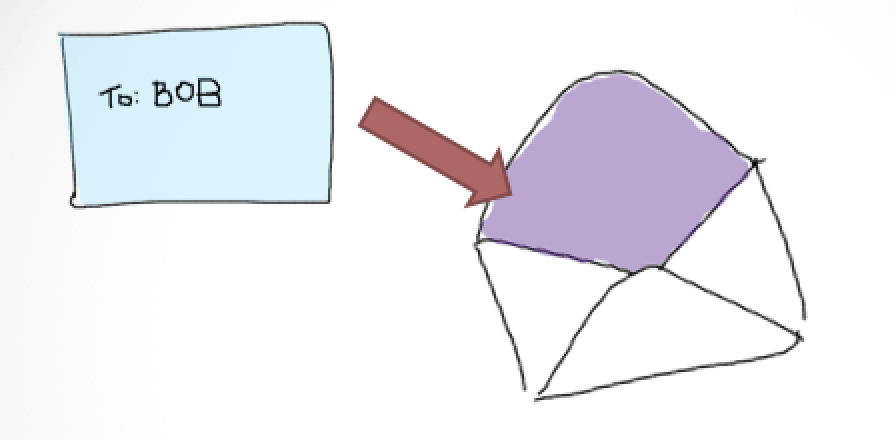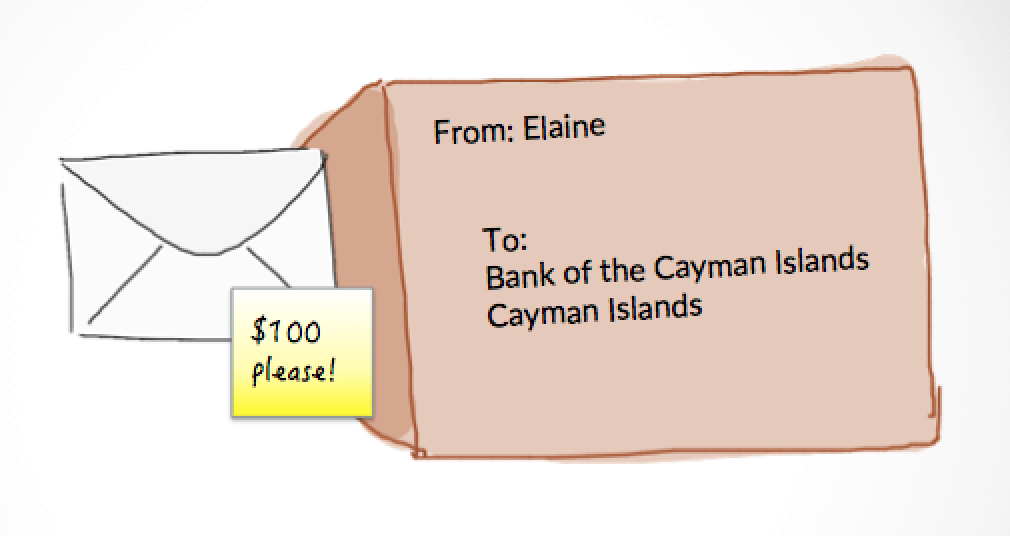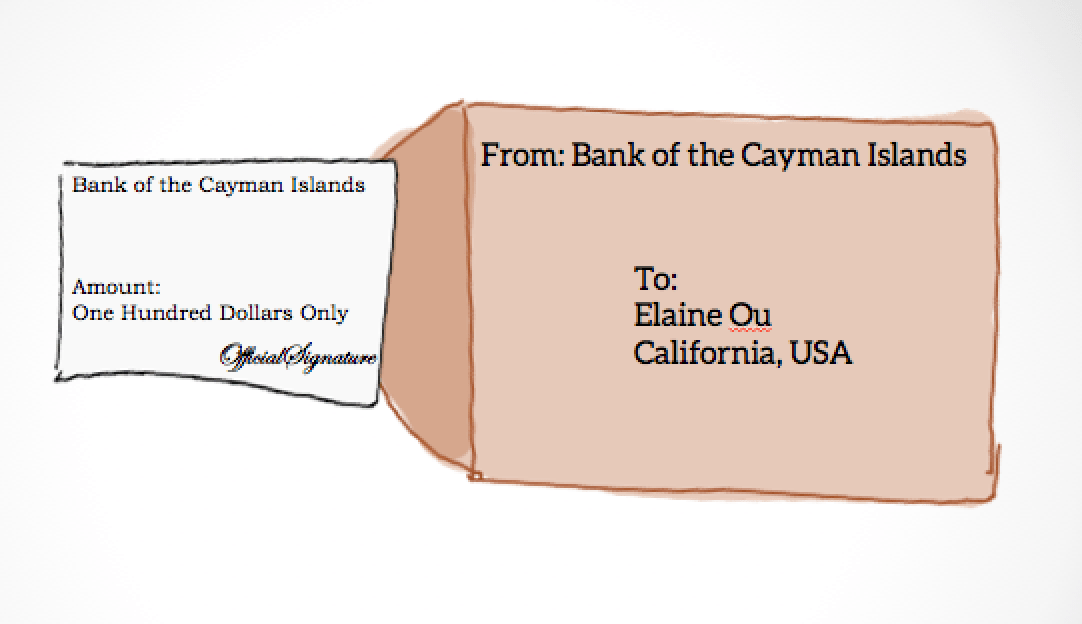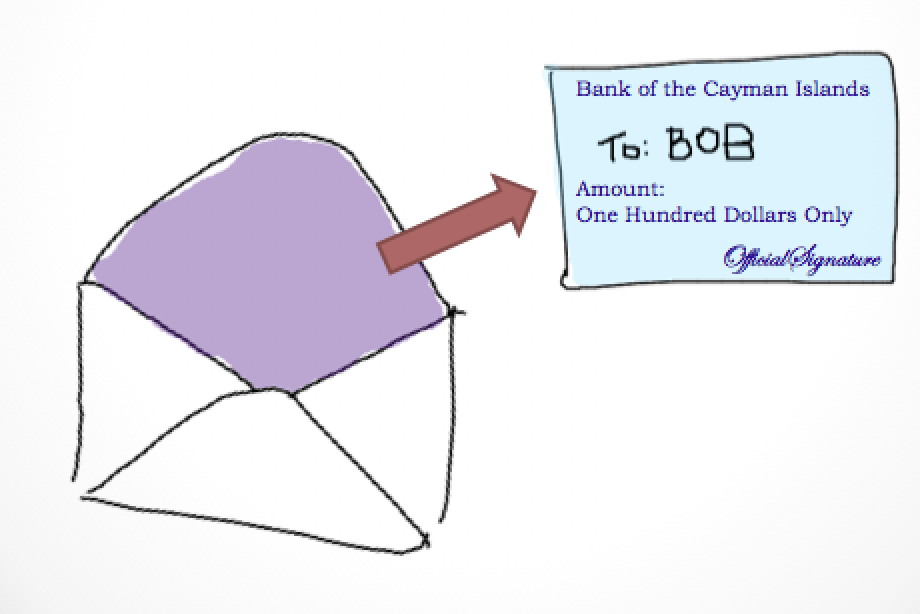# Zero-Knowledge Payment Protocols: DigiCash

Blind Demand Drafts

It’s 1962 and Elaine wants to transfer money to Bob. Bob lives in Cuba, which is under US embargo. Or maybe Bob is an unpopular political candidate, or whatever. The important thing is that the bank needs to clear the payment without seeing the name of the recipient, because Elaine doesn’t want a record of her association with Bob.Elaine writes Bob’s name on a slip of paper and puts it in a carbon-lined envelope. She seals the envelope and puts it in a bigger envelope along with a payment request of, say, $100. Elaine seals and signs the outer envelope, then mails the whole thing to her bank in the Cayman Islands.The bank opens Elaine’s outer envelope and finds the small envelope and withdrawal request inside. They deduct$100 from her account and print a signed demand draft on the outside of the little envelope without opening it. The bank puts the carbon envelope in a new outer envelope and sends it back to Elaine.Elaine receives the little envelope and forwards it to Bob.

Bob now has the carbon-lined envelope. He opens it and sees the original piece of paper with his name, now with the carbon copy of the bank’s signed demand draft imprint. Bob sends it to the bank, which processes tons of these every day. The bank doesn’t know that this demand draft was created by Elaine — it only recognizes that the demand draft has a valid bank signature, and credits Bob’s account with $100.Random Numbers But wait! Bob gets his hands on a ditto machine and makes copies of the demand draft before sending it to the bank. Now he can redeem the same payment over and over again. Before Elaine mails the original slip of paper to the bank, she has to generate a secret random number. The secret number is unique to each payment request. Assume Elaine has a quantum computer or something, so there’s no chance that anyone else could ever generate the same number.The bank keeps a running list of these numbers as each demand draft is paid out. The list is append-only, so a duplicate demand draft can’t be redeemed no matter how much time has passed. And that’s how a bank can authorize a blind payment without ever knowing that Elaine did business with Bob. DigiCash Now it’s 1994 and nobody uses snail mail anymore. Elaine wants to pay Bob$100, but let’s start by having Elaine pay Bob $1. Elaine once again generates a secret random number. Call it$x$. The job of the carbon-lined envelope is performed using a function$c$.$c(x)$ does not reveal the value of$x$, but it has the following property:$c^{-1}(S(c(x))) = S(x)$$S(c(x))$ is the bank’s signature on the outside of the carbon-lined envelope.$c^{-1}$ removes the effects of$c$ while allowing the signature to remain, just like when the carbon-lined envelope is removed. The payment process now looks like this: 1. Elaine sends the result of$c(x)$ to her bank. 2. The bank deducts$1 from her account and applies$S$ to create$S(c(x))$.
3. Elaine computes$c^{-1}(S(c(x))) = S(x)$, and sends$S(x)$ to Bob.
4. Bob forwards$S(x)$ to the bank and receives his dollar.

Bob can also forward$S(x)$ to the next person because$S(x)$ is now a bearer instrument. When$S(x)$ finally returns to the bank, it is added to the list of numbers the bank has already seen so that no one can spend it again.

Here,$S(x)$ is a single-denomination note. Digital signatures are cheaper than postage, so Elaine could repeat this process 100 times to send \$100.

Blind RSA signatures

If you haven’t fallen asleep yet, let’s define the functions. The simplest implementation is based on RSA signing.$\begin{array}{rclcll} c(x) & = & x' & \equiv & xr^e & \pmod N \\ S(c(x)) & = & S' & \equiv & (x')^d & \pmod N \\ c^{-1}(S(c(x))) & = & S & \equiv & S' \cdot r^{-1} & \pmod N \end{array}$$N$ and$e$ make up the bank’s public signature.$d$ is the bank’s private key.$r$ is a random blinding factor (it’s unrelated to random number$x$). RSA keys must satisfy the equation:$r^{ed} \equiv r \pmod N$

therefore:$S \equiv S' \cdot r^{-1} \equiv (x')^dr^{-1} \equiv x^dr^{ed}r^{-1} \equiv x^drr^{-1} \equiv x^d \pmod N$

Zcash

Now it’s 2016 and nobody trusts banks. We want to put zero-knowledge bearer instruments on the blockchain, but I have to go do other stuff so we’ll continue this another day.

References:
1. David Chaum, Blind Signatures for Untraceable Payments. Advances in Cryptology Proceedings of Crypto, 1982.
2. Nick Szabo, Contracts with Bearer. 1997